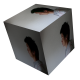# Finding genes with co-expression patterns

Can the R bioconductor package “WGCNA” find artefactually created modules?

Firstly some (subpar) code to generate an artefactual list of genes with co-expression patterns (modules):

```#!/usr/bin/perl

use strict;
use warnings;

my \$usage = "Usage \$0 <number of samples> <number of rows> <number of modules>\n";
my \$number_of_sample = shift or die \$usage;
my \$number_of_row = shift or die \$usage;
my \$number_of_module = shift or die \$usage;

my @master_pattern = ();

foreach my \$sample (1 .. \$number_of_sample){
my \$sample_name = 'sample' . \$sample;
}

my \$chunk = sprintf("%.0f",\$number_of_row / \$number_of_module);
for (my \$i = 1; \$i <= \$number_of_row; \$i += \$chunk){
#determine module pattern
my @pattern = ();
for (1 .. \$number_of_sample){
#randomly generate 0 or 1
my \$rand = int(rand(2));
push(@pattern,\$rand);
}
my \$pattern = "@pattern";
push(@master_pattern,\$pattern);
#go in chunks or modules
for (my \$j = \$i; \$j < \$i + \$chunk; ++\$j){
my \$p = join('',@pattern);
my \$expression = "\${j}_\$p\t";
foreach my \$pattern (@pattern){
my \$random_expression = random_expression(\$pattern);
\$expression .= "\$random_expression\t";
}
\$expression =~ s/\t\$//;
print "\$expression\n";
}
}

warn("Patterns:\n");
foreach my \$pattern (@master_pattern){
warn("\$pattern\n");
}

sub random_expression {
my (\$which) = @_;
my \$rand = '';
if (\$which == '0'){
\$rand = '1000';
while (\$rand >= 500){
\$rand = int(rand(1000));
}
} else {
\$rand = '0';
while (\$rand <= 500){
\$rand = int(rand(1000));
}
}
return \$rand;
}
```

Running the code:

./generate_random_module.pl 10 1000 20 > 10_sample_1000_list_20_module.tsv

Patterns:
1 0 0 0 0 1 0 0 0 1
0 0 0 0 0 0 1 0 1 1
0 0 1 0 0 0 1 0 0 1
1 0 0 0 0 1 1 1 0 1
0 1 0 0 1 0 0 1 1 0
0 0 0 0 1 1 1 1 0 0
1 1 1 0 0 0 0 0 1 0
1 1 1 0 0 0 0 0 0 0
1 1 1 1 1 1 1 1 0 1
0 0 1 1 1 0 1 1 1 0
0 1 1 0 0 0 0 0 1 1
0 1 0 0 1 0 0 1 1 0
0 0 1 1 0 0 1 0 1 0
0 0 0 0 1 1 1 1 0 1
0 1 0 0 1 1 0 0 0 1
1 0 0 1 1 1 0 1 1 0
0 1 1 1 1 1 1 0 1 0
0 1 0 1 0 0 1 1 1 0
0 1 1 0 1 0 1 0 0 0
0 1 1 1 0 1 1 0 1 1

So there are 20 artefactually created co-expression patterns (1 for over expression and 0 for under expression). Some patterns look very similar.

```library("WGCNA")

options(stringsAsFactors=FALSE)

test = as.data.frame(t(data[,-c(1)]))

names(test) = rownames(data)

rownames(test) = names(data)[-c(1)]

#gsg = goodSamplesGenes(test,verbose=3)

#gsg\$allOK

sampleTree = flashClust(dist(test),method="average")

png (filename="clustering.png", width=2000, height=2000, pointsize=12)
par(cex=0.6)
par(mar=c(0,4,2,0))
plot(sampleTree, main="Sample clustering", sub="", xlab="", cex.lab=1.5,cex.axis=1.5,cex.main=2)
abline(h=40000,col="red")
dev.off ()  ##  Close the image

#powers = c(c(1:10), seq(from=12,to=20,by=2))
#
#sft = pickSoftThreshold(test,powerVector=powers, verbose=5)
#
net = blockwiseModules(test,power=6,minModuleSize=30,reassignThreshold=0, mergeCutHeight=0.25,numericLabels=TRUE,pamRespectsDendro=FALSE,saveTOMs=TRUE,saveTOMFileBase="testTOM",verbose=3)
#
table(net\$colors)
#
#sizeGrWindow(12,9)
#
mergedColors = labels2colors(net\$colors)
#
png (filename="plot_dendro_and_colors.png", width=2000, height=2000, pointsize=12)
plotDendroAndColors(net\$dendrograms[], mergedColors[net\$blockGenes[]], "Module colors", dendroLabels= FALSE, hang=0.03, addGuide = TRUE, guideHang = 0.05)
dev.off()
#
moduleLabels = net\$colors
#
moduleColors = labels2colors(net\$colors)
#
MEs = net\$MEs

geneTree = net\$dendrograms[]

save(MEs, moduleLabels, moduleColors, geneTree, net, file = "number_2.RData")
```

A total of 15 modules are detected:

1 2 3 4 5 6 7 8 9 10 11 12 13 14 15
108 106 96 94 94 61 56 55 54 54 53 50 46 43 30Some of the patterns were very similar and were probably merged together, hence 15 out of the 20 artificial modules were detected. Also note that over expression was generated by randomly picking a number between 500 and 1000 and under expression was from 1 – 500. Looks pretty good!.
1.Zhuofei says:

Nice post! The textbox for the R script looks nice. I also want to directly insert the R script into Microsoft powerpoint or Word. I’m wondering how to generate such a textbox containing the R codes with the original font color and line number.

Zhuofei

1.Davo says:

Hi Zhuofei,

there are many tools to highlight the R syntax. Check out Rmarkdown and Rstudio. From there you can copy and paste it into Word or PowerPoint.

Cheers,

Dave

This site uses Akismet to reduce spam. Learn how your comment data is processed.